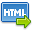Volume No. :   9

Issue No. :  4

Year :  2017

ISSN Print :  0975-4393

ISSN Online :  2349-2988Registration

## Ideals and Symmetrc Left Bi-Derivations on Prime Rings

 Address: Dr. C. Jaya Subba Reddy, G. Venkata Bhaskara Rao Department of Mathematics, Sri Venkateswara University, Tirupati-517502, Andhra Pradesh, India. *Corresponding Author
DOI No: 10.5958/2349-2988.2017.00102.4

ABSTRACT:
Let R be a non commutative 2, 3-torsion free prime ring and I be a non zero ideal of R. Let D(.,.):R×R?R be a symmetric left bi-derivation such that D(I,I)?I and d is a trace of D. If (i)[d(x),x]=0, for all x?I, (ii) [ d(x),x]?Z(R), for all x?I, then D=0. Suppose that there exists symmetric left bi-derivations D_1 (.,.):R×R?R and D_2 (.,.):R×R?R and B(.,.):R×R?R is a symmetric bi-additive mapping, such that (i) D_1 (d_2 (x),x)=0, for all x?I, (ii) d_1 (d_2 (x) )=f(x), for all x?I, where d_1 and d_2 are the traces of D_1 and D_2 respectively and f is trace of B, then either D_1=0 or D_2=0. If D acts as a left (resp. right) R-homomorphism on I, then D=0.
KEYWORDS:
Prime ring, Symmetric mapping, Trace, Bi-additive mapping, Symmetric bi-additive mapping, Symmetric bi-derivation, Symmetric left bi-derivation.
Cite:
C. Jaya Subba Reddy, G. Venkata Bhaskara Rao. Ideals and Symmetrc Left Bi-Derivations on Prime Rings. Research J. Science and Tech. 2017; 9(4): 601-604.[View HTML][View PDF]

Visitor's No. :   217838